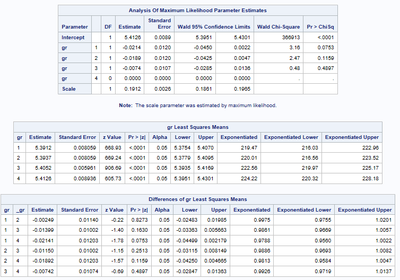## Geometric mean ratios with 95% confidence intervals

I have a dataset of IgG values for three groups. How can I compare the exponentiated differences between log10 transformed group means and present them as geometric mean ratios with associated 95% confidence intervals?
5 REPLIES 5

## Re: Geometric mean ratios with 95% confidence intervals

Examples of the data that you currently have would be helpful.

Geometric means normally involve the natural log. Are you sure that Log10 is needed?PGStats
Opal | Level 21

## Re: Geometric mean ratios with 95% confidence intervals

An example:

``````data heart;
set sashelp.heart;
lChol = log(Cholesterol);
label lChol="Log(Cholesterol)";
run;

proc genmod data=heart;
class smoking_status;
model lChol = smoking_status;
lsmeans smoking_status / diff exp cl;
run;``````
PG

## Re: Geometric mean ratios with 95% confidence intervals

I used Proc genmod on some dummy sas-data preparing for real data to calculate GMT, and GMT ratios. I want to compare groups 1-3 separately vs group 4 as regards the ratios and the results are below but I cannot find a way to output the lsmeans gr / diff exp cl results into a dataset for further processing (add to table outputs). Any suggestions? Also the procedure used for the  would be appreciated (guess I have to investigate the SAS documentation):

proc genmod data=gmt;
class gr;
model lChol = gr;
lsmeans gr / diff exp cl;
*output out=out_gmt ;
run;PGStats
Opal | Level 21

## Re: Geometric mean ratios with 95% confidence intervals

ods trace on;

before the procedure call to get a list of available output datasets in the log. Then use a statement such as

`ods output LSMeans=myLsmeans Diffs=myDiffs;`

among the procedure statements to output the datasets.

@HF_clin wrote:

... guess I have to investigate the SAS documentation...

definitely!

PG

## Re: Geometric mean ratios with 95% confidence intervals

Thanks! Definitely!
Discussion stats
• 5 replies
• 6592 views
• 2 likes
• 4 in conversation